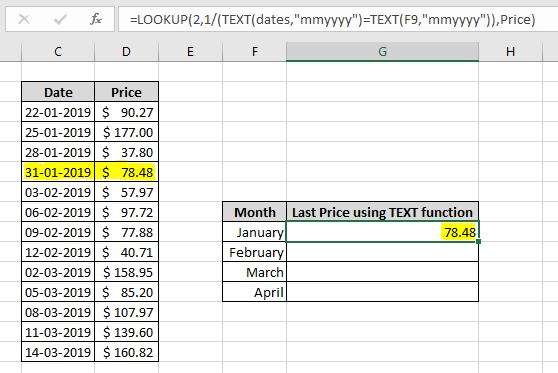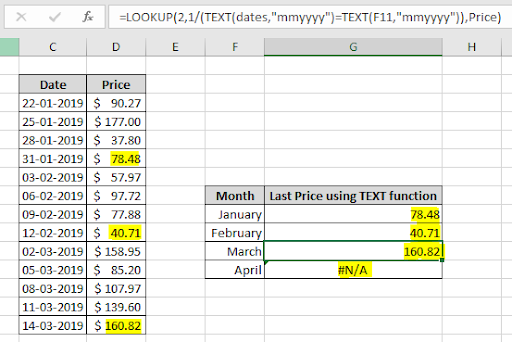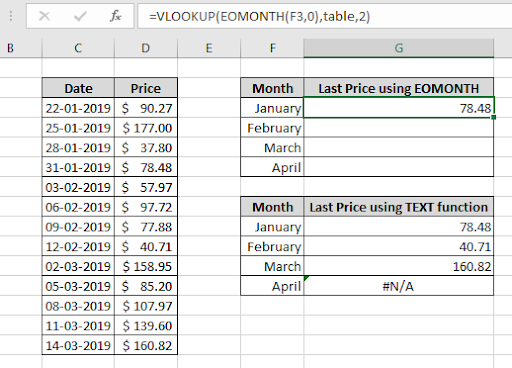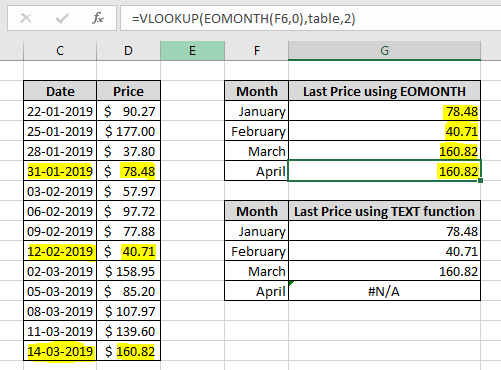# How to get last entry by month in ExcelIn this article, we will learn How to get the last entry by month in Excel.

Scenario:

When working with datasets, sometimes we need to extract some results based on different criteria. Here the problem criteria is the value must be the last entry corresponding to a given month. The problem can be interpreted in two ways.

1. Last entry in column by month
2. Last date value of month in column

You must be thinking what is the difference between the two. The difference is the first problem extracts the last entry from the column which matches the month name whereas the second problem extracts the nearest date value from the end of month date value. We will understand both situations one by one. Firstly we will be considering How to get the last entry in column by month.

How to get the Last entry in column by month in Excel?

For this we will be using the TEXT function and LOOKUP function. Here we use an attribute of LOOKUP function search for the last value. LOOKUP function takes 3 arguments lookup value, lookup array and result array. So we use the lookup array argument as 1/lookup array. For more details see the below formula syntax:

formula syntax :

 =LOOKUP(2,1/(TEXT(dates,"mmyyyy")=TEXT(month,"mmyyyy")),values)

dates : dates array in data

values : result array in data

month : month criteria

Example :

All of these might be confusing to understand. Let's understand how to use this formula in an example. Here we have data with date and Price values. For this we need the latest entry of January 2019 month which will be the last entry with date of Jan 2019. Here for the date array and Price array we used named ranges.

Use the formula:

 =LOOKUP(2,1/(TEXT(dates,"mmyyyy")=TEXT(F9,"mmyyyy")),Price)

Explanation:

• TEXT(dates,"mmyyyy") will return the array of values in "mmyyyy" format after converting it into text values which is

{ "012019" ; "012019" ; "012019" ; "012019" ; "022019" ; "022019" ; "022019" ; "022019" ; "032019" ; "032019" ; "032019" ; "032019" ; "032019" }

• TEXT(F9,"mmyyyy") will return the date value (in F9 cell) in "mmyyyy" format after converting the lookup date value which is "012019" and this value will be matched with the above stated array.
• Taking the division with 1 will convert the array of True values to 1s and False values to #DIV/0 error. So we will get the returned array as.

{ 1 ; 1 ; 1 ; 1 ; #DIV/0! ; #DIV/0! ; #DIV/0! ; #DIV/0! ; #DIV/0! ; #DIV/0! ; #DIV/0! ; #DIV/0! ; #DIV/0! }

• Now the LOOKUP function finds the value 2 in the array which will not be found. So it returns the entry corresponding to the last 1 value.Named ranges used

dates : C3:C15

Price : D3:D15

The last Price is 78.48 corresponding to the 31-01-2019. Copy the formula to other cells using the Ctrl + D or dragging down from the bottom right corner of the cell.As you can see the formula returns all the values required. The function returns #N/A error, if the lookup month value doesn't match any date entry. In the above example the date entries are in sorted manner. So the last entry of the month matches the last date entry of the month. Now if the date entries are not sorted, then we will first sort the date values and use the above formula.

How to get the Last date entry by month in column in Excel?

For this we will be using the VLOOKUP and EOMONTH function. The EOMONTH function takes the date value of month and returns the last date of the required month. VLOOKUP function finds the last date of month or the nearest previous date and returns a value corresponding to that value.

Use the formula :

 = VLOOKUP ( EOMONTH (F3,0), table, 2)

Explanation :

1.  EOMONTH (F3,0) returns the last date of the same month. 0 argument used to return the date from the same month.
2. Now the lookup value becomes the last date of the given month.
3. Now the value will be searched in the first column of table and look for the last date, if found returns the value corresponding to value and if not found then it returns the last matching result, nearest to the lookup value and returns its corresponding result.
4. The function returns the value from the 2nd column of the table as 3rd argument is 2.Here the formula returns the 78.48 as result. Now copy the formula to other cells using the shortcut Ctrl + D or dragging down from the right bottom corner of the cell.As you can see the formula works fine. There is just one problem. As we look up the last date corresponding to the april month, the function returns the value corresponding from the March, as there is no entry of april date in table. Make sure to catch these results.

Here are all the observational notes regarding using the formula.

Notes :

1. Use the formula corresponding to the problem stated in the article.
2. VLOOKUP and LOOKUP function are functions when lookup value is number and not present in the lookup array, returns the value corresponding to just the nearest number smaller than the lookup value.
3. Excel stores date values as numbers.
4. The above two stated functions return only one value.
5. Use the 4th argument in VLOOKUP function as 0 to get the exact match of the lookup value in the table.

Hope this article about How to get the last entry by month in Excel is explanatory. Find more articles on extracting values from the table using formulas here. If you liked our blogs, share it with your friends on Facebook. And also you can follow us on Twitter and Facebook. We would love to hear from you, do let us know how we can improve, complement or innovate our work and make it better for you. Write us at info@exceltip.com

Related Articles:

Find the last row with mixed data in Excel : working with numbers, text or blank cell in the same array. Extract the last row with non blank cell using the MATCH function in Excel.

Finding the Last Day of a Given Month : EOMONTH function returns the last date of the month, of the given date value.

How to Get Last Value In Column : Last entry in a large data, A combination of CELL and INDEX function returns the last value in a column or list of data.

Difference with the last non blank cell : takes the alternate difference from the last value in the list with numbers using the IF and LOOKUP function in Excel.

Find the last row of data with text values in Excel : In an array of text values and blank cells, return the last value in the array using the MATCH and REPT function in excel.

How to use the SUMPRODUCT function in Excel: Returns the SUM after taking the product of the corresponding values in multiple arrays in excel.

How to use wildcards in excel : Lookup, Count, sum, average and more mathematical operation on cells matching phrases using the wildcards in excel.

Popular Articles:

How to use the IF Function in Excel : The IF statement in Excel checks the condition and returns a specific value if the condition is TRUE or returns another specific value if FALSE.

How to use the VLOOKUP Function in Excel : This is one of the most used and popular functions of excel that is used to lookup value from different ranges and sheets.

How to use the COUNTIF Function in Excel : Count values with conditions using this amazing function. You don't need to filter your data to count specific values. Countif function is essential to prepare your dashboard.

How to use the SUMIF Function in Excel : This is another dashboard essential function. This helps you sum up values on specific conditions.

Terms and Conditions of use

The applications/code on this site are distributed as is and without warranties or liability. In no event shall the owner of the copyrights, or the authors of the applications/code be liable for any loss of profit, any problems or any damage resulting from the use or evaluation of the applications/code.